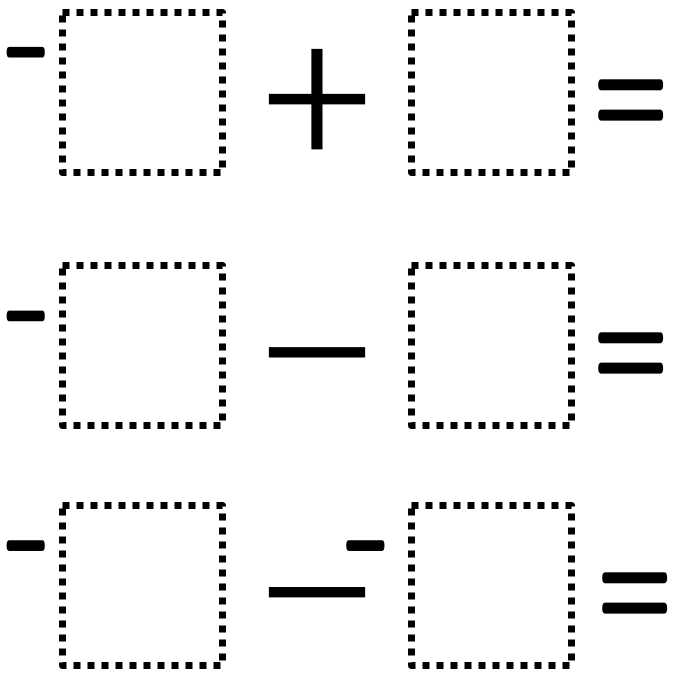Directions: Using the digits 1 to 6, at most one time each, fill in the boxes so that top two equations are equal and the bottom equation has the greatest value.### Hint

If you want the first two answers to be the same, should they both be positive or negative?
What pairs of numbers from 1-6 can you combine to make the same outcome?
How could we rewrite the second problem?
How could we rewrite the third problem?

First possible solution:
-6 + 3 = -3
-2 – 1 = -3 (2 and 1 in any order)
-4 – -5= 1 (or -5 – -4 = -1)

Second possible solution:
-6 + 1 = -5
-2 – 3 = -5 (2 and 3 in any order)
-4 – -5 = 1 (or -5 – -4 = -1)

Third possible solution:
-6 + 2 = -4
-1 – 3 = -4 (1 and 3 in any order)
-4 – -5 = 1 (or -5 – -4 = -1)

Source: Kate Nerdypoo

## Sides of a Triangle

Directions: The perimeter of a triangle is 20 units. Using whole numbers, how many sets …

1.Another one:

-6+2=-1-3=-4
and -4<-4-(-5)

•Great spot Dominique – I have added your suggestion to the list of solutions.

2.-6 + 5 = -1
-4 – (make it addition) 3 = -1
-2 – (make it addition) -1 (make positive) = -1
would also work

3.4.5.Sub 2 dogey63 on yt

6.-1 wouldn’t be the greatest possible value, would it?